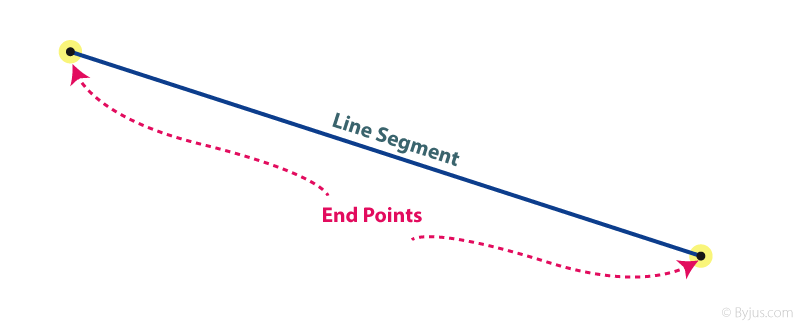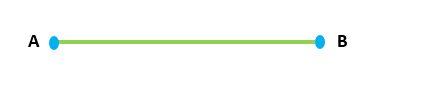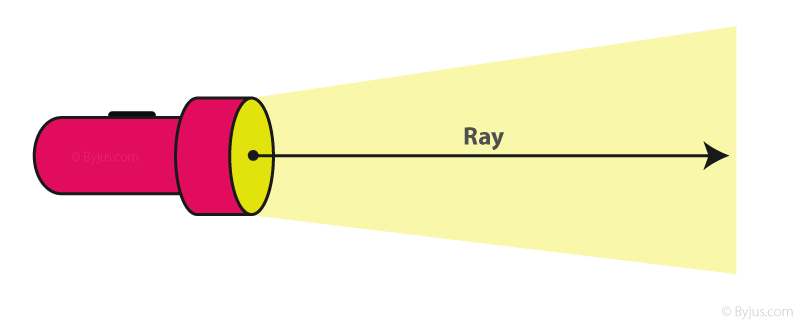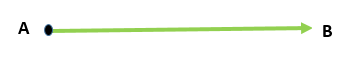# Line Segment And Ray

We come across many shapes in daily life. Consider a triangle, a square or any other shape. To draw any of these figures, one begins with a line or a line segment or a curve. Depending upon the number and arrangement of these lines we get distinct types of shapes and figures. For example, a triangle is a figure enclosed by 3 line segments, Pentagon is a polygon bounded by 5 line segments and so on. We have already discussed line now let us see what is line segment and ray.

## What is a Line Segment?A line segment is a part of a line having two endpoints. Figures such as a triangle, polygon, hexagon, square are made of different numbers of line segments. The measure of a line segment is called its length.  In contrast to the infinitely extending line, a line segment has a fixed length and can be measured easily. A line segment with A and B as two endpoints is represented as $\overline{AB}$.## What is a Ray?Ray is another part of a line. It is a combination of a line and a line segment that has an infinitely extending end and one terminating end. As its one end is non-terminating, its length cannot be measured. A ray is represented by $\overrightarrow{AB}$  where one end is symbolized by an endpoint and the infinitely extending part by an arrow.To solve problems on the topic, download BYJU’S – The Learning App from Google Play Store and watch interactive videos. Also, take free tests to practice for exams. To study other topics, visit www.byjus.com and browse among thousands of interesting articles.

## Frequently Asked Questions on Line Segment and Ray

### What is a line segment?

The line segment is a part of a line having two endpoints. The distance between the two endpoints can be measured and hence they can form the sides of any polygon.

### What is a ray?

A ray is a part of the line having one fixed point and the other point does not have an end. It means that a ray has one terminating end and the other end is extending infinitely.

### What is the difference between a line segment and a ray?

A line segment is a part of a line having two endpoints and its length is measurable. Whereas a ray has one fixed point (starting point), but it has no endpoint. So, its length cannot be measurable.

### What is the difference between line and line segment?

A line has no endpoints and can be extended on both sides. Whereas, a line segment has two endpoints and cannot be extended on both sides.

### Can we extend the line segment?

No, the line segment cannot be extended as it has two endpoints and a definite length. Whereas, we can extend the line in two opposite directions and a ray can be extended in one end.

Quiz on Line segments and ray

1. Laurea